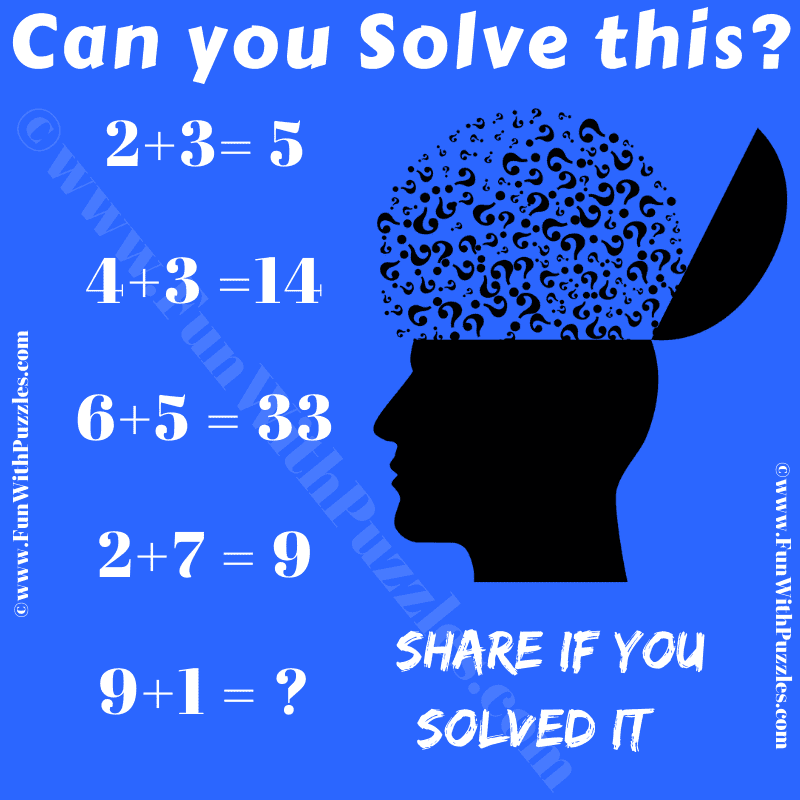This is a very interesting puzzle that will test your maths skills and logical reasoning. In this, you will be shown some logical number equations. These number equations may not make any sense mathematically. However, there is logical code that can be applied to these equations to make these equations logical correct. Can you crack this logical hidden code and find the value of the missing number in the last number equation?Can you solve this Math and Logic Puzzle?

The answer to this "Math and Logic Puzzle", can be viewed by clicking on the answer button.